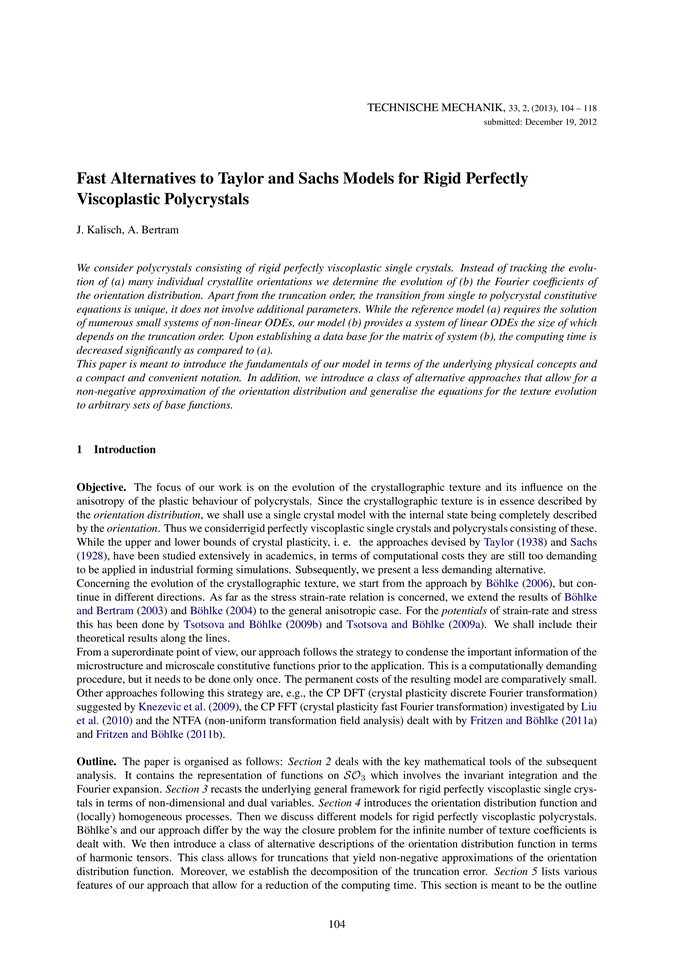# Fast Alternatives to Taylor and Sachs Models for Rigid Perfectly Viscoplastic Polycrystals

• J. Kalisch
• A. Bertram

## Abstract

We consider polycrystals consisting of rigid perfectly viscoplastic single crystals. Instead of tracking the evolution of (a) many individual crystallite orientations we determine the evolution of (b) the Fourier coefficients of the orientation distribution. Apart from the truncation order, the transition from single to polycrystal constitutive equations is unique, it does not involve additional parameters. While the reference model (a) requires the solution of numerous small systems of non-linear ODEs, our model (b) provides a system of linear ODEs the size of which depends on the truncation order. Upon establishing a data base for the matrix of system (b), the computing time is decreased significantly as compared to (a).

This paper is meant to introduce the fundamentals of our model in terms of the underlying physical concepts and a compact and convenient notation. In addition, we introduce a class of alternative approaches that allow for a non-negative approximation of the orientation distribution and generalise the equations for the texture evolution to arbitrary sets of base functions.